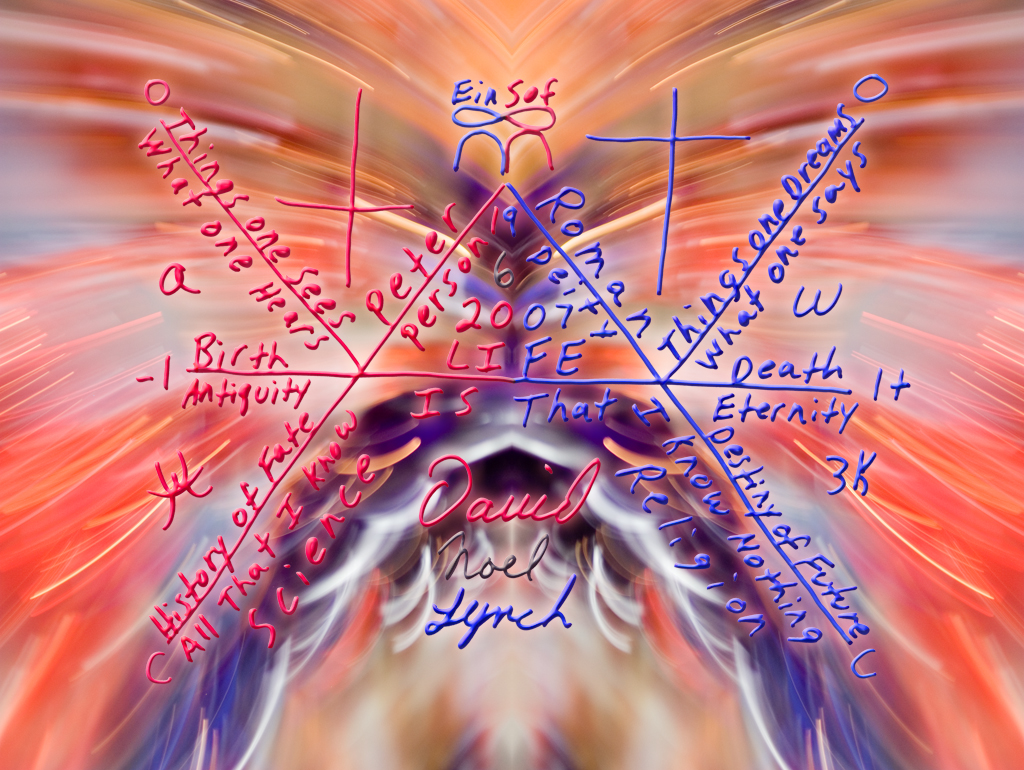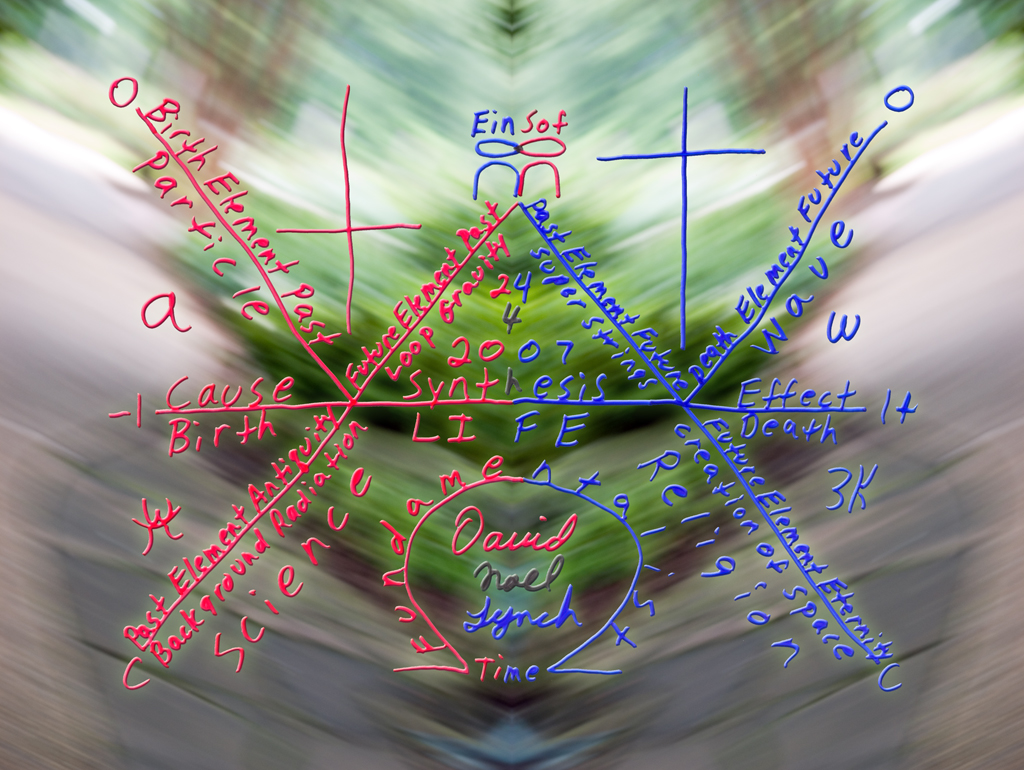Date: Tue, 20 May 2008 02:49:43 -0700 (PDT)
From: "David Lynch" <dnl1960 at yahoo.com>
Subject: Nancy Ellen Abrams
CC: "Nancy Ellen Abrams" <cosmicuroboros at yahoo.com>, "Joel R. Primack" <joel at ucsc.edu>

Israel,

I found a link to your site on an Abrams web page. You make a statement,
"T=0K =-273,160C. The philosophy of science begins from this point. The physics begins from this point.
The religion begins with this temperature. The origin of Existence begins with this temperature. "

Thus the boundary of existence is between absolute zero and the speed of light.
However; it could be said that the philosophy of science begins at zero K, the realm of religion begins at the speed of light,
and the origin of Existence begins at some point in the middle.

For example: using Einstein's equation, energy is equal to mass times the speed of light squared,
we know the amount of energy that is contained in any object that crosses the zero degree Kelvin line.
E=mc^2=Existence..

Since light is both particle and wave, using the same analogy we can say that Science is the particle and Religion is the wave.
At any moment of time we can express Einstein's equation as E=cmc=Existence.

What would happen to current models if Einstein would have created an equation for energy that said, ((P(+l+w+h)c)*(F(-l-w-h)c))
Read as, ((Past times mass times the speed of light) times (Future times inverted mass times the speed of light)).

Particle, a, alpha, in the past just under the speed of light, and Wave, w, omega, in the future just over the speed of light.
The only time that E=cmc holds true is at the infinite of a moment. The realm of existence. A Triangulum of time.

I have created an equation for a moment in time that uses the energy of Einstein, the force of Newton, and the logic of Socrates.
I call it the KnoWell.http://www.lynchphoto.com/avignon.html

In the KnoWell model, inner space is bubbling out clockwise through quantum loops of gravity,
while outer space is collapsing in counter clockwise through super strings of symmetry.http://www.lynchphoto.com/wreck.html

Thus dark matter could be just under zero degrees Kelvin while dark energy is just above the speed of light.
My theory throws a curve ball at the theory of the big bang. Putting us smack dab in the middle of a steady state universe.
The collapse of outer space could explain why the stars appear to be red shifted. Making gravity a push not a pull.

Below is a link to a video of me drawing a KnoWell.